Tamilnadu State Board New Syllabus Samacheer Kalvi 8th Maths Guide Pdf Chapter 1 Numbers InText Questions Text Book Back Questions and Answers, Notes.

## Tamilnadu Samacheer Kalvi 8th Maths Solutions Chapter 1 Numbers InText Questions

Recap Exercise (Text Book Page No. 3)

Question 1.
The simplest form of $$\frac{125}{200}$$ is
$$\frac{125}{200}=\frac{125 \div 25}{200 \div 25}=\frac{5}{8}$$
= $$\frac{5}{8}$$Question 2.
Which of the following is not an equivalent fraction of $$\frac{8}{12}$$ ?
(A) $$\frac{2}{3}$$
(B) $$\frac{16}{24}$$
(C) $$\frac{32}{60}$$
(D) $$\frac{24}{36}$$
(C) $$\frac{32}{60}$$
$$\frac{8}{12}=\frac{8+4}{12 \div 4}=\frac{2}{3}$$
$$\frac{8}{12}=\frac{8 \times 2}{12 \times 2}=\frac{16}{24}$$
$$\frac{8}{12}=\frac{8 \times 3}{12 \times 3}=\frac{24}{36}$$
But $$\frac{32}{60}=\frac{32 \div 5}{60 \div 5}=\frac{6.4}{12}$$
∴ $$\frac{32}{60}$$ is not equivalent fraction of $$\frac{8}{12}$$

Question 3.
Which is bigger $$\frac{8}{9}$$ or $$\frac{4}{5}$$ ?
LCM of 5 and 9 = 45$$\frac{8}{9}$$ is bigger than $$\frac{4}{5}$$The LCM of 12 and 16 is 48.

Question 4.
Add the fractions : $$\frac{3}{5}+\frac{5}{8}+\frac{7}{10}$$.
LCM of 5, 8, 10 = 5 × 2 × 4
= 40Question 5.
Simplify: $$\frac{1}{8}-\left(\frac{1}{6}-\frac{1}{4}\right)$$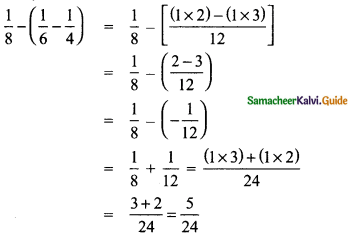Question 6.
Multiply: $$2 \frac{3}{5}$$ and $$1 \frac{4}{7}$$.
$$2 \frac{3}{5} \times 1 \frac{4}{7}=\frac{13}{5} \times \frac{11}{7}=\frac{143}{35}=4 \frac{3}{35}$$

Question 7.
Divide $$\frac{7}{36}$$ by $$\frac{35}{81}$$.
$$\frac{7}{36}+\frac{35}{81}=\frac{7}{36} \times \frac{81}{35}=\frac{9}{20}$$

Question 8.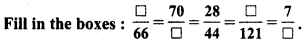Question 9.
In a city $$\frac{7}{20}$$ of the population are women and $$\frac{1}{4}$$ are children. Find the fraction of the population of men.
Let the total population = 1
Population of men = Total population – Women – Children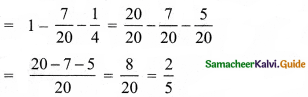∴ Population of men = $$\frac{2}{5}$$

Question 10.
Represent $$\left(\frac{1}{2}+\frac{1}{4}\right)$$ by a diagram.Try These (Text Book Page No. 3)

Question 1.
Is the number -7 a rational number? Why?
A rational number, Because – 7 = $$\frac{-14}{2}=\frac{p}{q}$$

Question 2.
Write any 6 rational numbers between 0 and 1.
$$\frac{1}{2}, \frac{1}{3}, \frac{1}{4}, \frac{1}{5}, \frac{1}{6}, \frac{1}{7}$$

Try These (Text Book Page No. 4)

That’s literally all there is to it! 1/8 as a decimal is 0.125.

Write the decimal forms of the following rational numbers:

Question 1.
$$\frac{4}{5}$$
$$\frac{4}{5}=\frac{4 \times 20}{5 \times 20}=\frac{80}{100}=0.80$$

Question 2.
$$\frac{6}{25}$$
$$\frac{6}{25}=\frac{6 \times 4}{25 \times 4}=\frac{24}{100}=0.24$$5/9 as a decimal is 0.56

Question 3.
$$\frac{486}{1000}$$
$$\frac{486}{1000}$$ = 0.486

Question 4.
$$\frac{1}{9}$$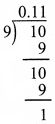$$\frac{1}{9}$$ = 0.11….

Question 5.
$$3 \frac{1}{4}$$$$3 \frac{1}{4}$$ = $$\frac{13}{4}$$ = 3.25

That’s literally all there is to it! 5/8 as a decimal is 0.625.

Question 6.
$$-2 \frac{3}{5}$$$$-2 \frac{3}{5}$$ = $$\frac{-13}{5}$$ = -2.6Try These (Text Book Page No. 6)

Question 1.
$$\frac{7}{3}=\frac{?}{9}=\frac{49}{?}=\frac{-21}{?}$$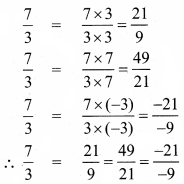Question 2.
$$\frac{-2}{5}=\frac{?}{10}=\frac{6}{?}=\frac{-8}{?}$$Try These (Text Book Page No. 7)

Question 1.
Which of the following pairs represents equivalent rational numbers?
(i) $$\frac{-6}{4}, \frac{18}{-12}$$
(ii) $$\frac{-4}{-20}, \frac{1}{-5}$$
(iii) $$\frac{-12}{-17}, \frac{60}{85}$$
(i) $$\frac{-6}{4}, \frac{18}{-12}$$
$$\frac{-6}{4}=\frac{-6 \times 3}{4 \times 3}=\frac{-18}{12}$$
∴ $$\frac{-6}{4}$$ equivalent to $$\frac{-18}{12}$$

(ii) $$\frac{-4}{-20}, \frac{1}{-5}$$
$$\frac{-4}{-20}=\frac{-4 \div(-4)}{-20 \div(-4)}=\frac{1}{5} \neq-\frac{1}{5}$$
∴ $$\frac{-4}{-20}$$ equivalent to $$\frac{1}{-5}$$

(iii) $$\frac{-12}{-17}, \frac{60}{85}$$
$$\frac{-12}{-17}=\frac{-12 x-5}{-17 x-5}=\frac{60}{85}$$
∴ $$\frac{-12}{-17}$$ equivalent to $$\frac{60}{85}$$Question 2.
Find the standard form of
(i) $$\frac{36}{-96}$$
(ii) $$\frac{-56}{-72}$$
(iii) $$\frac{27}{18}$$
(i) $$\frac{36}{-96}$$
= $$\frac{-36 \div 12}{96 \div 12}=\frac{-3}{8}$$

(ii) $$\frac{-56}{-72}$$
= $$\frac{-56 \div(-8)}{-72 \div(-8)}=\frac{7}{9}$$

(iii) $$\frac{27}{18}$$
= $$1 \frac{9}{18}=1 \frac{1}{2}$$

Question 3.
Mark the following rational numbers on a number line.
(i) $$\frac{-2}{3}$$
$$\frac{-2}{3}$$ lies betveen 0 and -1.
T?ìe unit part between O and —lis divided into 3 equal parts and second part is taken.(ii) $$\frac{-8}{-5}$$
$$\frac{-8}{-5}$$ = $$1 \frac{3}{5}$$
$$1 \frac{3}{5}$$ lies between I and 2, The unit part between I and 2 is divided into 5 equal parts and the third part is taken.(iii) $$\frac{5}{-4}$$
$$\frac{5}{-4}$$ = $$-\frac{5}{4}$$ = $$-1 \frac{1}{4}$$
$$-1 \frac{1}{4}$$ lies between -1 and -2. The unit part between -1 and -2 is divided into four equal parts and the first part is taken.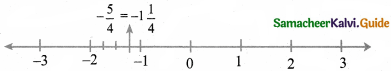Think (Text Book Page No. 15)

Is zero a rational number? If so, what is its additive inverse
Yes zero a rational number Additive inverse of zero is zero.

Think (Text Book Page No. 16)

What is the multiplicative inverse of 1 and -1?
Multiplicative inverse of 1 is 1 and -1 is -1.

Try These (Text Book Page No. 16)

Divide
(i) $$\frac{-7}{3}$$ by 5
(ii) 5 by $$\frac{-7}{3}$$
(iii) $$\frac{-7}{3}$$ by $$\frac{35}{6}$$
(i) $$\frac{-7}{3}$$ by 5(ii) 5 by $$\frac{-7}{3}$$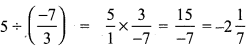(iii) $$\frac{-7}{3}$$ by $$\frac{35}{6}$$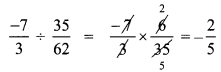Try These (Text Book Page No. 20)

The closure property on integers holds for subtraction and not for division. What about rational numbers? Verify.
Let 0 and $$\frac{1}{2}$$ te two rational numbers 0 – $$\frac{1}{2}$$ = –$$\frac{1}{2}$$ is a rational numter
∴ Closure property for subtraction holds for rational numbers.
But consider the two rational number $$\frac{5}{2}$$ and 0.
$$\frac{5}{2}$$ + 0 = $$\frac{5}{2 \times 0}=\frac{5}{0}$$
Here denominator = 0 and it is not a rational number.
∴ Closure property is not true for division of rational numbers.

Try These (Text Book Page No. 22)

(i) Is $$\frac{3}{5}-\frac{7}{8}=\frac{7}{8}-\frac{3}{5}$$ ?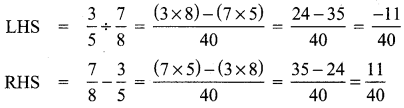LHS ≠ RHS
∴ $$\frac{3}{5} \div \frac{7}{8}$$ ≠ $$\frac{7}{8}-\frac{3}{5}$$
∴ Subtraction of rational numbers is not commutative.

(ii) $$\frac{3}{5} \div \frac{7}{8}=\frac{7}{8} \div \frac{5}{3}$$? So, what do you conclude?LHS ≠ RHS
∴ $$\frac{3}{5} \div \frac{7}{8}$$ ≠ $$\frac{7}{8} \div \frac{5}{3}$$
∴ Commutative property not hold good br division of rational numbers.Try This (Text Book Page No. 22)

Check whether associative property holds for subtraction and division.
Consider for rational numbers $$\frac{2}{3}, \frac{1}{2}$$ and $$\frac{3}{4}$$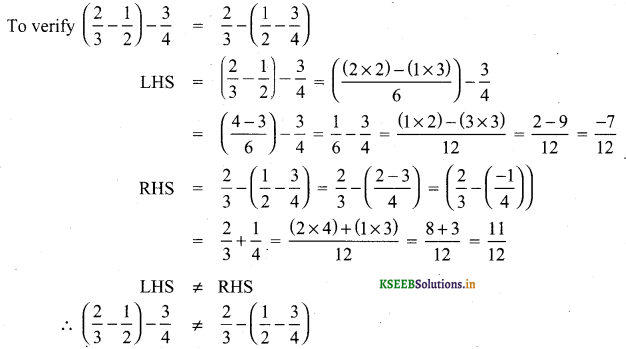∴ Associative property not holds for subraction of rational numbers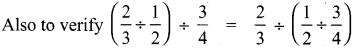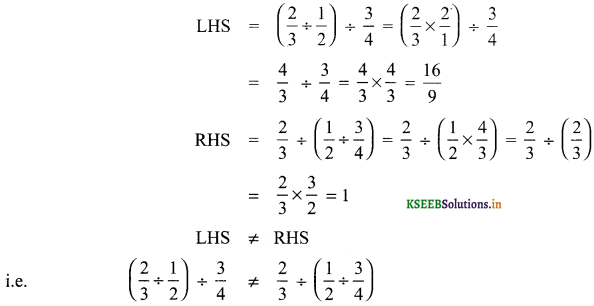∴ Associative property not holds for division of rational numbersTry This (Text Book Page No. 25)

Question 1.
Observe that,
$$\frac{1}{1.2}+\frac{1}{2.3}=\frac{2}{3}$$
$$\frac{1}{1.2}+\frac{1}{2.3}+\frac{1}{3.4}=\frac{3}{4}$$
$$\frac{1}{1.2}+\frac{1}{2.3}+\frac{1}{3.4}+\frac{1}{4.5}=\frac{4}{5}$$
Use your reasoning skills, to find the sum of the first 7 numbers in the pattern given above.Think (Text Book Page No. 26)

Question 1.
Is the square of a prime number, prime?
No, the square of a prime number ‘P’ has at Least 3 divisors 1, P and P2. But a prime number is a number which has only two divisors, 1 and the number itself. So square of a prime number is not prime.

Question 2.
Will the sum of two perfect squares always be a perfect square? What about their difference and their product?
The sum of two perfect squares, need not be always a perfect square. Also the difference of two perfect squares need not be always a perfect square. Bu the product of two perfect square is a perfect square.

Try These (Text Book Page No. 26)

Question 1.
Which among 256, 576, 960, 1025,4096 are perfect square numbers? (Hint: Try to extend the table of squares already seen).
256 = 162
576 = 242
4096 = 642
∴ 256, 576, and 4096 are perfect squaresQuestion 2.
One can judge just by look that each of the following numbers 82, 113, 1972, 2057, 8888, 24353 is not a perfect square. Explain why?
Because the unit digit ola perfect square will be 0, 1,4, 5, 6, 9. But the given numbers have unit digits 2, 3, 7, 8. So they are not perfect squares.

Think (Text Book Page No. 27)

Consider the claim: “Between the squares of the consecutive numbers n and (n + 1), there are 2n non-square numbers’ Can it be true? FInd how many non-square numbers are there
(i) between 4 and 9 ?
(ii) between 49 and 64? and Verify the claim.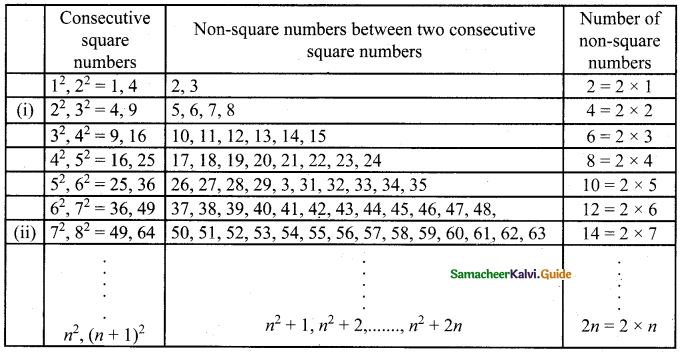Therefore we conclude that there are 2n non-square numbers between two consecutive square numbers.

Think (Text Book Page No. 30)

In this quick guide we’ll describe what the factors of 96 are, how you find them and list out the factor pairs of 96 for you to prove the calculation works.

In this case, if we want to find the smallest factor with which we can multiply or divide 108 to get a square number, what should we do?
108 = 2 × 2 × 3 × 3 = 22 × 32 × 3
If we multiply the factors by 2, then we get
22 × 32 × 3 × 3 = 22 × 32 × 32 = (2 × 3 × 3)2
Which is perfect square.
∴ Again if we divide by 3 then we get 22 × 32 ⇒ (2 × 3)2, a perfect square.
∴ We have to multiply or divide 108 by 3 to get a perfect square.Try These (Text Book Page No. 32)

Find the square root by long division method.
Question 1.
400√400 = 20

Question 2.
1764√1764 = 42Question 3.
9801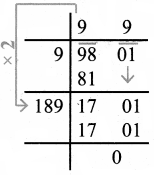√9801 = 66

Try These (Text Book Page No. 32)

without calculating the square root, guess the number of digits in the square root of the following numbers:
Question 1.
14400
$$\sqrt{14400}$$ = $$\sqrt{144 \times 100}$$
= $$\sqrt{144} \times \sqrt{100}$$
= 12 × 10
= 120

Question 2.
390625
$$\sqrt{390625}$$ = $$\sqrt{25 \times 25 \times 25 \times 25}$$
= $$\sqrt{25 \times 25} \times \sqrt{25 \times 25}$$
= 25 × 25
= 625Question 3.
100000000
$$\sqrt{100000000}$$ = $$\sqrt{10000 \times 10000}$$
= $$\sqrt{10000} \times \sqrt{10000}$$
= 100 × 100
= 10,000

Try These (Text Book Page No. 33)

Find the square root of
Question 1.
5.4756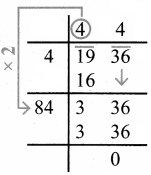Question 2.
19.36Question 3.
116.64Think (Text Book Page No. 33)

Try to fill in the blanks using √ab = √a × √b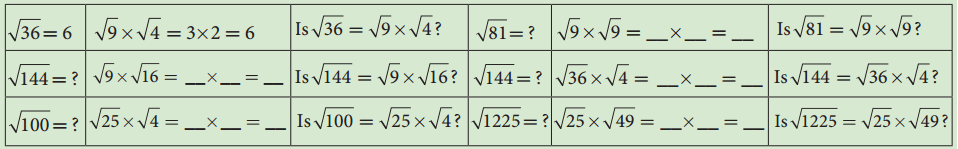Try These (Text Book Page No. 34)

Using this method, find the square root of the numbers 1.2321 and 11.9025.
(i) 1.2321
√1.2321 = $$\sqrt{\frac{12321}{10000}}$$
= $$\frac{111}{100}$$ = 1.11

(ii) 11.9025
√11.9025 = $$\frac{\sqrt{119025}}{\sqrt{10000}}$$
= $$\frac{345}{100}$$ = 3.45

Try These (Text Book Page No. 34)

Write the numbers in ascending order.
Question 1.
4, √14, 5
Squaring all the numbers we get 42, (√14)2, 52 ⇒ 16, 14, 25
∴ Ascending order: 14, 16, 25
Ascending order: √14, 4, 5

Question 2.
7, √65, 8
Squaring 7, √65 and 8 we get 72, (√65)2, 82 ⇒ 49, 65, 64
Ascending order : 49, 64, 65
Ascending order: 7, 8, √65Try These (Text Book Page No. 37)

Find the ones digit in the cubes of each of the following numbers.
(i) 12
(ii) 27
(iii) 38
(iv) 53
(v) 71
(vi) 84
(i) 12
12 ends with 2, so its cube ends with 8 i.e. ones digit in 123 is 8.

(ii) 27
27 ends with 7, so its cube end with 3. i.e., ones digit in 273 is 3.

(iii) 38
38 ends with 8, so its cube ends with 2 i.e. ones digit in 383 is 2.

(iv) 53
53 ends with 3, so its cube ends with 7. i.e, ones digit in 533 is 7.

(v) 71
71 ends with 1, so its cube ends with 1. i.e. ones digit in 713 is 1

(vi) 84
84 ends with 4, so its cube ends with 4. i.e, ones digit in 843 is 4.Try These (Text Book Page No. 41)

Expand the following numbers using exponents:
(i) 8120
(ii) 20,305
(iii) 3652.01
(iv) 9426.521
(i) 8120
8120 = (8 × 1000) + (1 × 100) + (2 x×10) + 0 × 1
= (8 × 103) + (1 × 102) + (2 × 101)

(ii) 20,305
20305 = (2 × 10000) + (0 × 1000) + (3 × 100) + (0 × 10) + (5 × 1)
= (2 × 104) + (3 × 102) + 5

(iii) 3652.01
3652.01 = 3000 + 600 + 50 + 2 + $$\frac{0}{10}+\frac{1}{100}$$
= (3 × 1000) + (6 × 100) + (5 × 10) + (2 × 1) + (1 × $$\frac{1}{100}$$)
= (3 × 103) + (6 × 102) + (5 × 101) + 2 + (1 × 10-2)

(iv) 9426.521
= (9 × 1000) + (4 × 100) + (2 × 10) + (6 × 1) + $$\left(\frac{5}{10}\right)+\left(\frac{2}{100}\right)+\left(\frac{1}{1000}\right)$$
= (9 × 103) + (4 × 102) + (2 × 101) + 6 + (5 × 10-1) + (2 × 10-2) + (1 × 10-3)Try These (Text Book Page No. 42)

Verify the following rules (as we did above). Here, a,b are non-zero integers and are any integers.
1. Product of same powers to power of product rule: am × bm = (ab)m
2. Quotient of same powers to power of quotient rule: $$\frac{a^{m}}{b^{m}}=\left(\frac{a}{b}\right)^{m}$$
3. Zero exponent rule: a0 = 1.
let a = 2; b = 3; m = 2
1. am × bm = 22 × 32
= 4 × 9 = 36 = (2 × 3)2

2. $$\frac{a^{m}}{b^{m}}=\frac{2^{2}}{3^{2}}=\frac{4}{9}=\left(\frac{2}{3}\right)^{2}$$

3. a0 = 20 = 1.

Try These (Text Book Page No. 44)

Question 1.
Write in standard form: Mass of planet Uranus is 8.68 × 1025 kg.
Mass of Planet Uranus = 86800000000000000000000000 kg
[23 zeros after 88]

Question 2.
Write in scientific notation:
(i) 0.000012005